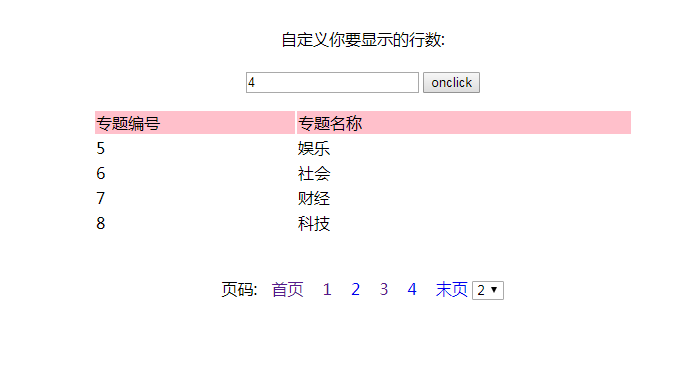javaweb 用户可以自由选择出现的行列数(每页要显示多少)；``````<%@ page contentType="text/html;charset=UTF-8"%>
<%@ page import="cn.jbit.pagination.dao.PageDao" %>
<%@ page import="cn.jbit.pagination.bean.Topic" %>
<%@ page import="java.util.List" %>
<%

String pageNumber = request.getParameter("pageNumber"); //显示第几页
String getNumber=request.getParameter("inp");
if(getNumber== null ||getNumber.equals("")){
getNumber="4";
}
if(pageNumber == null || pageNumber.equals("")){ //如果没有此参数为空

pageNumber = "1"; //缺省显示第一页

}
int number = Integer.parseInt(pageNumber); //转成整数类型
int RECORD_COUNT = Integer.parseInt(getNumber); //每页显示的数据条数

int start = 0; //从第几条开始

int end = 0; //到第几条结束
start = (number - 1) * RECORD_COUNT;
end = number * RECORD_COUNT;
System.out.println("start:"+start);
System.out.println("end:"+end);
PageDao dao = new PageDao();
List<Topic> topics = dao.list(start,end);
int count = dao.totalCount(); //总条数

int total = (count - (count%RECORD_COUNT))/RECORD_COUNT; //总页数

if(count % RECORD_COUNT != 0){
total++;
}
System.out.println("++"+getNumber);
System.out.println("++RECORD_COUNT"+RECORD_COUNT);

%>
<html>
<script language='Javascript'>
function selectShow(){
var getnumber=document.getElementById("select");
var getvalue=document.getElementById("select").value;
document.location='index.jsp?pageNumber='+getvalue+'&getNumber=<%= getNumber%>';
}
{
var getnumber=document.getElementById("select");
getnumber.selectedIndex = <%=pageNumber%> - 1;  //下拉菜单的索引号
}
function on(){
var getvalue=document.getElementById("input").value;
document.location='index.jsp?pageNumber=1&getNumber='+getvalue;

}

</script>
<title>航班查询</title>
<style>
a{text-decoration:none;}
</style>
<br/>

<center>

<form action="index.jsp?pageNumber=1&getNumber=<%=getNumber%>" method="post"><br/>
<input name="inp" id="input" value="<%= RECORD_COUNT%>"/>
<input type="submit" value="onclick" name="but" onclick="on()"/>
</form>
<table width='40%'  >
<tr bgcolor='pink'>
<td width='30%'  >专题编号</td>
<td width='50%'>专题名称</td>
</tr>
<%for(int i = 0;i < topics.size();i++){ %>
<tr>
<td width='30%'><%=topics.get(i).getTid() %></td>
<td width='50%'><%=topics.get(i).getTname() %></td>
</tr>
<%} %>
</table>
<br/>
<br/>

<a href="index.jsp?pageNumber=1&getNumber=<%=getNumber%>" >&nbsp;&nbsp;首页</a>&nbsp;&nbsp;&nbsp;
<%for(int i = 1;i <= total;i++) {%>
<a href="index.jsp?pageNumber=<%=i%>&getNumber=<%=getNumber%>"><%=i%></a>&nbsp;&nbsp;&nbsp;
<%}%>
<a href="index.jsp?pageNumber=<%=total%>&getNumber=<%=getNumber%>">末页</a>
<select id="select" onchange="selectShow()">
<%for(int i = 1;i <= total;i++) {%>
<option value="<%=i%>" ><%=i%></option>

<%}%>
</select>
</center>
</body>
</html>
``````

2个回答javaweb 用户可以自由选择出现的行列数(每页要显示多少)；
![图片说明](https://img-ask.csdn.net/upload/201903/31/1554038333_26492.png)rnrnrn我输入自己想要的行列数，比如5行，他会变成5行，但是如果再点下面的页码就又变成原来的显示行数rn```rn<%@ page contentType="text/html;charset=UTF-8"%>rn<%response.setHeader("Pragma","No-cache"); %>rn<%response.setHeader("Cache-Control","no-cache"); %>rn<%response.setHeader("Expires","0");%>rn<%@ page import="cn.jbit.pagination.dao.PageDao" %>rn<%@ page import="cn.jbit.pagination.bean.Topic" %>rn<%@ page import="java.util.List" %>rn<%rnrn String pageNumber = request.getParameter("pageNumber"); //显示第几页rn String getNumber=request.getParameter("inp");rn if(getNumber== null ||getNumber.equals(""))rn getNumber="4";rn rn if(pageNumber == null || pageNumber.equals("")) //如果没有此参数为空rnrn pageNumber = "1"; //缺省显示第一页rnrn rn int number = Integer.parseInt(pageNumber); //转成整数类型rn int RECORD_COUNT = Integer.parseInt(getNumber); //每页显示的数据条数rnrn int start = 0; //从第几条开始rnrn int end = 0; //到第几条结束rn start = (number - 1) * RECORD_COUNT;rn end = number * RECORD_COUNT;rn System.out.println("start:"+start);rn System.out.println("end:"+end);rn PageDao dao = new PageDao();rn List topics = dao.list(start,end);rn int count = dao.totalCount(); //总条数rnrn int total = (count - (count%RECORD_COUNT))/RECORD_COUNT; //总页数rnrn if(count % RECORD_COUNT != 0)rn total++;rn rn System.out.println("++"+getNumber);rn System.out.println("++RECORD_COUNT"+RECORD_COUNT);rn rn %>rnrnrn rn 航班查询rnrn rnrn rnrnrn自定义你要显示的行数: rn rnrnrn rn rn rn 专题编号rn 专题名称rnrn<%for(int i = 0;i < topics.size();i++) %>rn rn <%=topics.get(i).getTid() %>rn <%=topics.get(i).getTname() %>rnrn<% %>rnrn rn rn页码:rn 首页 rn<%for(int i = 1;i <= total;i++) %>rn<%=i%> rn<%%>rn末页rnrn<%for(int i = 1;i <= total;i++) %>rn <%=i%>rnrn<%%>rnrnrnrnrn```
[加急]怎样得到每页可显示多少行、 每页可以打印多少行 ?
rn 要打印 25 条记录 . 报表里每页可放 24 条记录 。 这样的话第二张纸就rnrn只有一行记录. ( 太浪费纸了 )rnrn 如果能动态得到每页多少行的话。 我就可以算出最后一页是多少行。 然后再决定是否压缩成一页了。rnrn 另: 不知有人做过这种压缩页的功能吗 ? 我想是 先判断最后一页的行数,决定是否压缩。 是的话再改变一下行高就行了， 一点也不难。 对吗 ？rnrn注：每页可显示多少行 和 每页可以打印多少行 是不同的。 每页可打印多行好像难一点

DataGridView无法自由选择行
winforms中，DataGridView控件在绑定数据源后默认选中了第一行，而且无法自由选择其他行了，是设置了什么属性吗

datagridview 最多可以显示多少行？
datagridview 最多可以显示多少行？

CRichEditCtrl最多显示多少行？

Product表，字段如下rnproductid(产品ID) productname（产品名） userid（发布用户）updatetime(更新时间)rn这张表中有1W多条数据，现在要求显示出所有的产品，使用分页的方法来显示（每页10条），但是每一页不能出现同一用户发布的两个产品。rn我用想使用group by userid来分组，发现每一个用户只有了一个产品了，这样他的其它产品就没有显示了。rn大家是如何解决这样的问题的？

C/C++:对于二维数组 a[m][n]：总数=sizeof（a）/sizeof（类型）列=sizeof（a）/sizeof(类型) 行=总数/列Vector:vector自带size属性int row=matrix.size();//行数int collor=matrix.size();//列数Java:java中的数组自带length属性a.length就是行数，a.leng...

getElementById().rows.length或者其他元素，反正不可以用jq对象，只能用js对象

js控制每页输出表格的多少行的问题、

reporting service 问题,每页多少行不能控制.

rdlc 打印模板 如何固定每页打印多少行
ActiveReports怎样设限定每页固定多少行?

TStringGrid如何按数据多少自动确定行列数

Sql2000 从多少行取到多少行

RT
listview可以显示多少列多少行数据？

excel 最多列数为多少？？？？
excel 最多列数为多少？？？？
QuickReport 怎么可以控制每页的显示数量？

MSHFlexGrid 最多可以有多少行

RT，由于ID字段有可能不连续，想查询行数之间的行rnrn不要临时表的那种

Launcher3 定制桌面行列数

jxl返回正确的行列数
// 返回去掉空行的记录数 private static int getRightRows(Sheet sheet) { int rsCols = sheet.getColumns(); // 列数 int rsRows = sheet.getRows(); // 行数 int nullCellNum; int afterRows = rsRows; for

[code=HTML] rn rn rn clickmern rn rn rn rn rn rn rn rn [/code]rn function clickA() rn var td = this.parent;rn alert(td.tagName);rn rntable的单元格里是anchor，点这个anchor(或其它控件)，如何得到当前行列？
msflexgrid最多能够显示多少行?
msflexgrid最多能够显示多少行?

Delphi 显示结果有多少行

C# DataTable最多显示多少行？
[color=#FF0000]C# DataTable最多显示多少行？ Winform中。[/color]rn今天 批量操作数据库，读取所有数据看看，只能显示出2000多条，但是我判断DataTable的行数，却是和数据库里面的一样。rnrn断点调试的时候，视图显示也就2000多行，我要查看所有的数据，该怎么弄？是不是DataTable只能显示这多，还是有什么设置的，rnrnrn[color=#FF0000]另外access数据库最多显示多少行呢？ [/color]rn"2007 access 文件共享锁定数溢出,修改 MaxLocksPerFile"，[color=#FF0000]我已经修改成50000了，为什么还是提示这个问题呢？[/color]